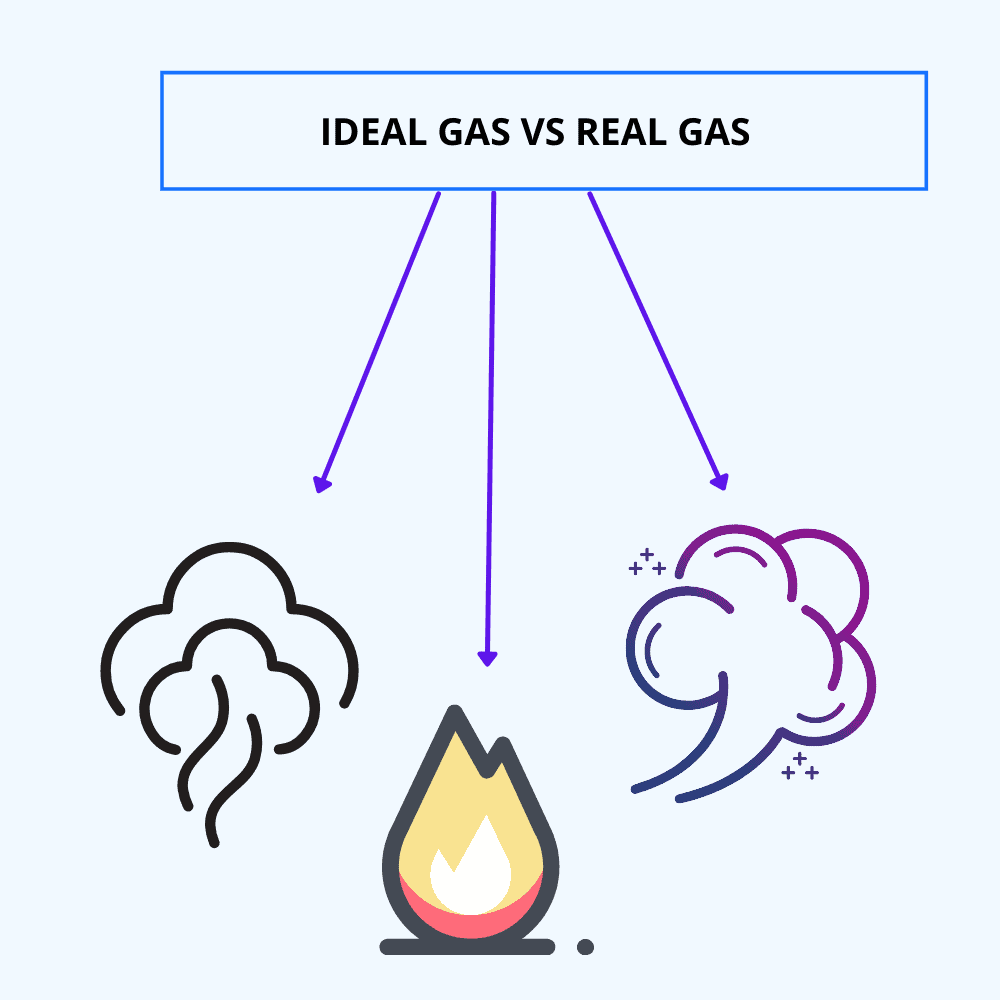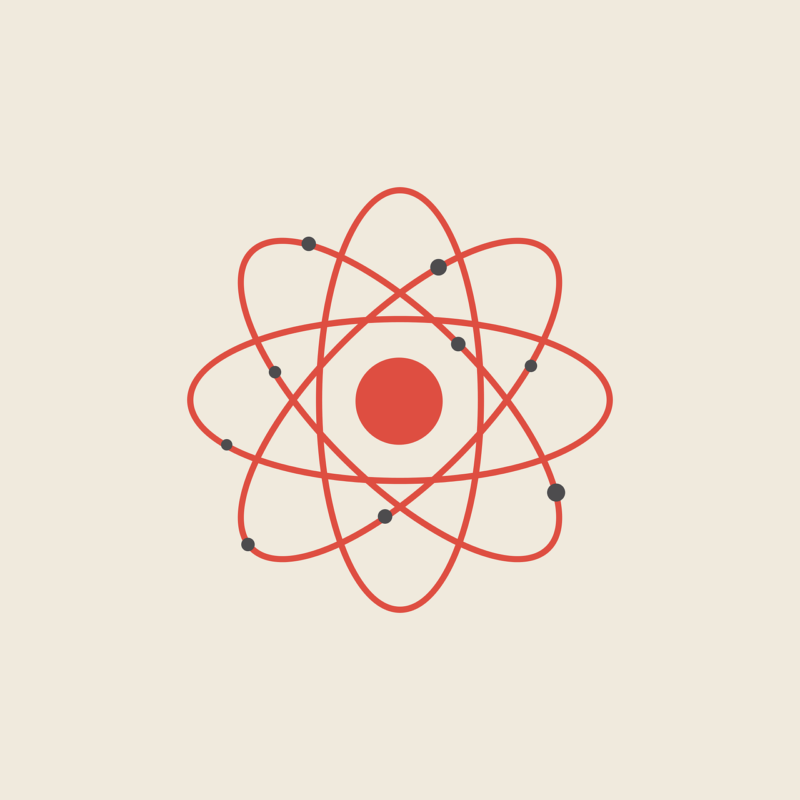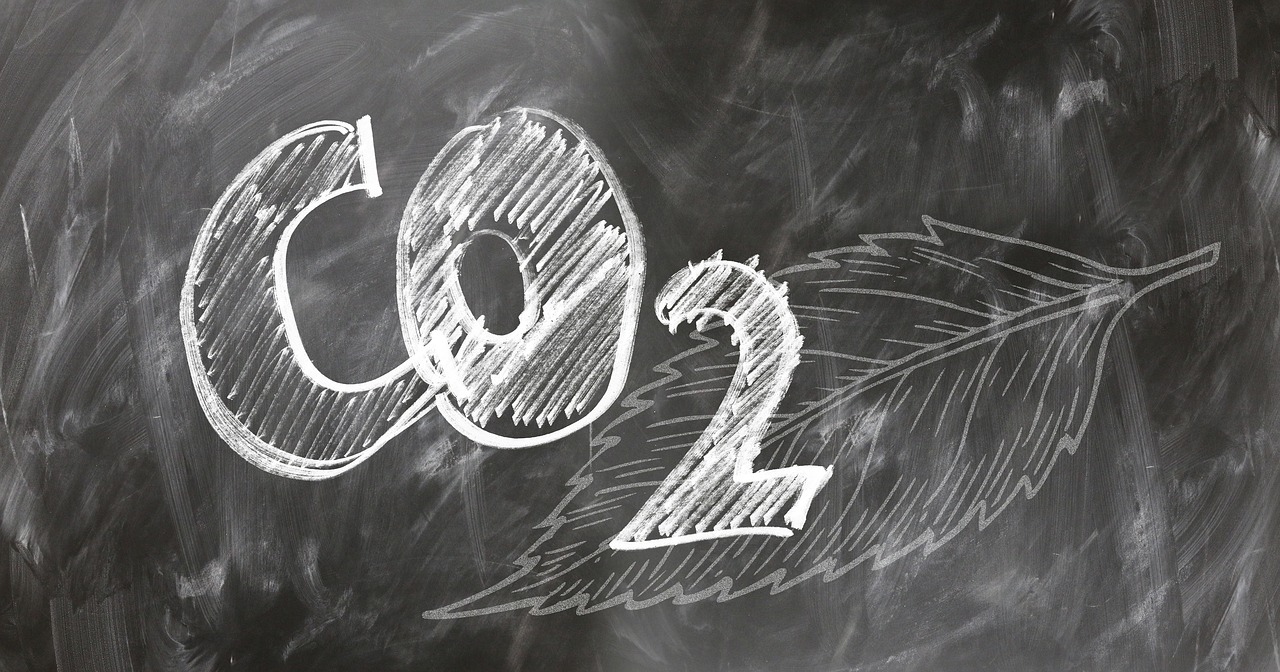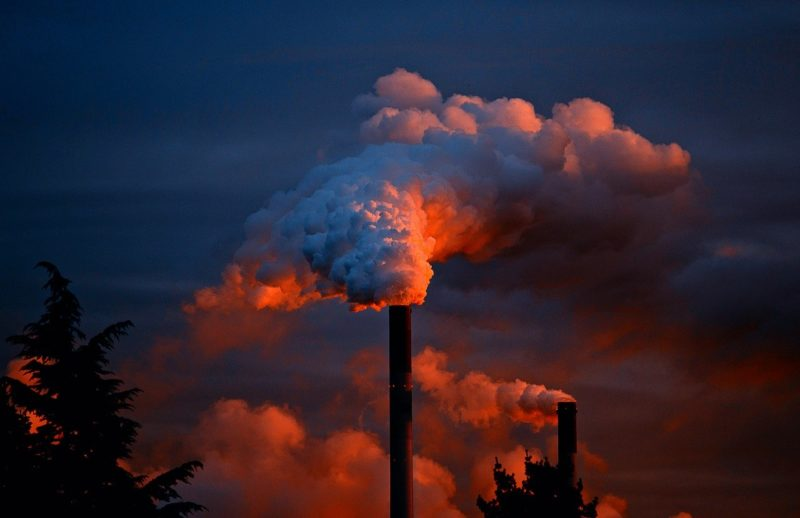# Ideal Gas vs Real Gas: Differences and Concept

Gas is a matter of small density that has no volume or shape of its own but adapts to the bowl, container, or flask where it is kept.

Gases are made up of atoms and molecules that interact with each other through intermolecular forces and occupy a finite volume.

Gases are made up of atoms and molecules that interact with each other through intermolecular forces and occupy a finite volume.

By the principles of pressure, volume and temperature it is possible to distinguish the real gas from the ideal gas.## Main differences between ideal gas and real gas

 Ideal gas Real Gas The ideal gas obeys all gas laws under all conditions of pressure and temperature. The real gas obeys the gas laws only under conditions of low pressure and high temperature. They obey Vanderwaal’s real gas equation. The volume occupied by the molecules is negligible with respect to the total volume. The volume occupied by the molecules is not negligible with respect to the total volume. There are no intermolecular forces of attraction. There are forces of attraction or repulsion between particles. It is a theoretical gas. It exists in the surrounding nature. It has a high pressure. Furthermore, it has a pressure correction term in its equation, and the actual pressure is lower than that of the ideal gas. PV = nRT (P+an2V2) * (V-nb) = nRT The molecules collide with each other elastically. Molecules collide with each other inelastically.
1. Main differences between ideal gas and real gas
2. An ideal gas is a hypothetical gas, while the real gas exists in the nature around us
3. The ideal gas obeys the gas laws and, on the other hand, real gases obey the gas laws under low pressure and high-temperature conditions.
4. The ideal gas does not undergo condensation, while real gases undergo condensation when cooled to their boiling point.
5. An ideal gas has a negligible volume compared to the total volume, while a real gas has a significantly larger volume compared to the ideal gas.
6. The ideal gas does not exist, while examples of real gas are oxygen, hydrogen, carbon, etc.
7. In an ideal gas, there are no intermolecular forces. In contrast, the intermolecular forces in a real gas can be repulsive or attractive.

## The ideal gasThe ideal or perfect gas is from the group of theoretical gases whose randomly moved point particles do not interact with each other, such as oxygen, hydrogen, and carbon dioxide; it also considers inversely proportional changes between pressure and volume, but directly proportional changes of both pressure and volume with respect to temperature.

On the other hand, the thermodynamic behavior of real gases does not follow the ideal gas equation, since it maintains high pressure and low temperature, i.e., it has very large density values.

The thermodynamic behavior of real gases does not follow the ideal gas equation, since it maintains high pressure and low temperature, i.e., it has very large density values.

According to the kinetic theory of gases, the behavior of an ideal gas is based on two premises: the molecules of gases are not point-like, and the interaction energy is not negligible.In the ideal gas model, substances are always in gaseous mode. More complex is the behavior of the real substance, which can undergo a phase or state change.

This phase change makes the isotherms of the real gas more complex than the isotherms of the ideal gas.

## The real gas

A real gas cannot be compressed indefinitely, contrary to the ideal gas hypothesis.

The real gas

At low pressures, and under identical circumstances, real gases are more compressible than ideal gases, but that happens when their pressure values are higher, which depends on the temperature and the type of gas.

Under normal conditions, when their chemical formula is simple and their relativity low, the pressure, and temperature of the real gases will equal those of the ideal gases, as is the case with helium.

The properties of the real gas cannot be explained by the ideal gas law; to understand them, compressibility effects, calorific capacity, non-equilibrium thermodynamic effects, and Van der Waals forces must be taken into account.

When we talk about Van der Waals forces, named after the Dutch scientist who won the Nobel Prize for Physics in 1910 and who investigated gases, we are referring to the repulsive and attractive forces between molecules that are very small in the case of real gases.

## Differences between real gas and ideal gas according to Van der WaalsAccording to the scientist, the difference between real gases and ideal gases is that one corrects the volume and the other modifies the pressure. He argues that real gases, at pressures and temperatures close to ambient, act like ideal gases.

Scientist says:

Van der Waals’ Law can be defined as an equation of state about the pressure of the gas, the universal constant of gases, the volume occupied by the gas, and its temperature in absolute value.

Van der Waals’ Law.

The equations of the real gases must be applied when measuring the behavior of a gas that differs from the usual conditions of an ideal gas.These equations show that real gases do not have infinite expansion, because if they did, they could not occupy a greater volume.

Finally, the ideal gas equation goes well when the intermolecular attraction of the gas is negligible and the molecules do not occupy a significant part of the total volume, which is true when the pressure is low and the temperature is high.

In other situations, the ideal gas law might give different results. But what is clear is that gases do not always behave as ideal gases.

Latest posts by Roberto (see all)